## Thursday, April 9, 2015

### Bayes factors for tests of mean and effect size can be very different

In this post, we consider a Bayes factor null-hypothesis test of the mean in a normal distribution, and we find the unintuitive result that the Bayes factor for the mean can be very different than the Bayes factor for the effect size. The reason is that the prior distribution of the standard deviation affects the implied prior on the effect size. Different vague priors on the standard deviation can dramatically change the BF on the effect size.

By contrast, the posterior distributions of the mean and of the effect size are very stable despite changing the vague prior on the standard deviation.

Although I caution against using Bayes factors (BFs) to routinely test null hypotheses (for many reasons; see Ch. 12 of DBDA2E, or this article, or Appendix D of this article), there might be times when you want to give them a try. A nice way to approximate a BF for a null hypothesis test is with the Savage-Dickey method (again, see Ch. 12 of DBDA2E and references cited there, specifically pp. 352-354). Basically, to test the null hypothesis for a parameter, we consider a narrow region around the null value and see how much of the distribution is in that narrow region, for the prior and for the posterior. The ratio of the posterior to prior probabilities in that zone is the BF for the null hypothesis.

Consider a batch of data randomly sampled from a normal distribution, with N=43. We standardize the data and shift them up by 0.5, so the data have a mean of 0.5 and an SD of 1.0. Figure 1, below, shows the posterior distribution on the parameters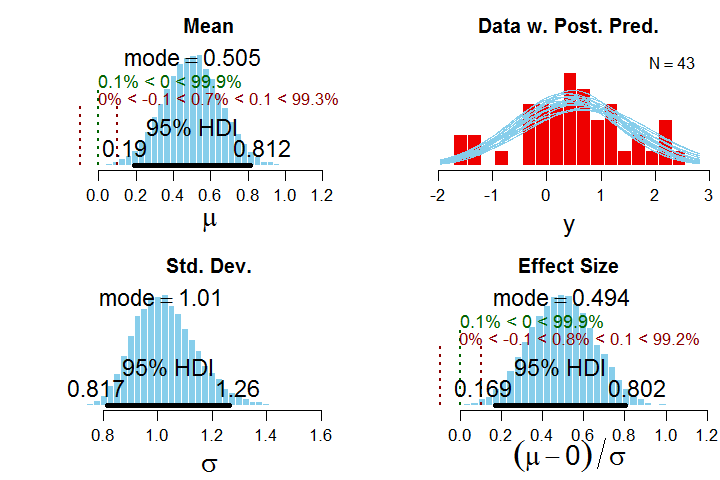Figure 1. Posterior when using unif(0,1000) prior on sigma, shown in Fig's 2 and 3.
First, consider the mu (mean) parameter. From the relation of the 95% HDI and ROPE, we would decide that a value of 0 for mu is not very credible, with the entire HDI outside the ROPE and only 0.7% of the posterior distribution practically equivalent to the null value. For the effect size, a similar conclusion is reached, with the 95% HDI completely outside the ROPE, and only 0.8% of the posterior practically equivalent to the null value. Note that the ROPEs for mu and effect size have been chosen here to be commensurate.

To determine Bayes factors (BFs) for mu and effect size, we need to consider the prior distribution in more detail. It has a broad normal prior on mu with an SD of 100 and a broad uniform prior on sigma from near 0 to 1000, as shown in Figures 2 and 3: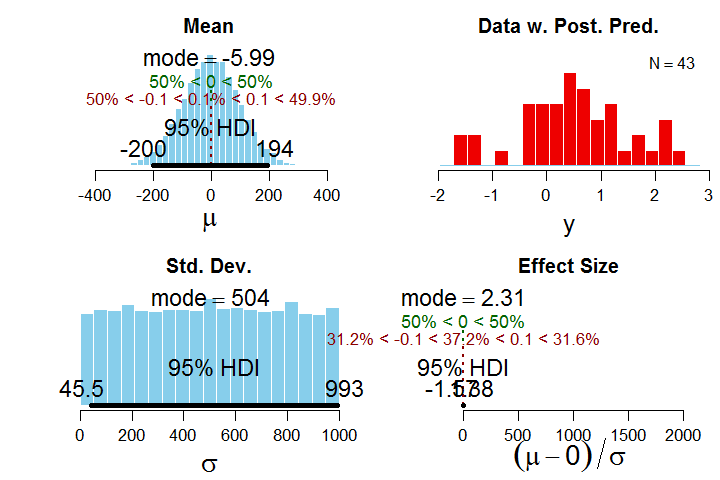Figure 2. Prior with unif(0,1000) on sigma.  Effect size is shown better in Figure 3.

The implied prior on the effect size, in the lower right above, is plotted badly because of a few outliers in the MCMC chain, so I replot it below in more detail: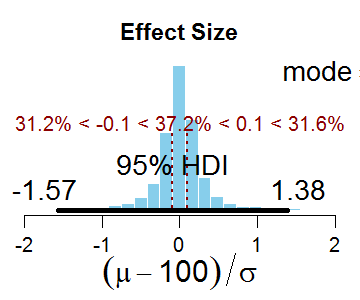Figure 3. Implied prior on effect size for unif(0,1000) prior on sigma.

The BF for a test of the null hypothesis on mu is the probability mass inside the ROPE for the posterior relative to the prior. In this case, the BF is 0.7% / 0.1% (rounded in the displays) which equals about 7. That is, the null hypothesis is 7 times more probable in the posterior than in the prior (or, more carefully stated, the data are 7 times more probable under the null hypothesis than under the alternative hypothesis). Thus, the BF for mu decides in favor of the null hypothesis.

The BF for a test of the null hypothesis on the effect size is the analogous ratio of probabilities in the ROPE for the effect size. The BF is 0.8% / 37.2% which indicates a strong preference against the null hypothesis. Thus, the BF for mu disagrees with the BF for the effect size.

Now we use a different vague prior on sigma, namely unif(0,10), but keeping the same vague prior on mu: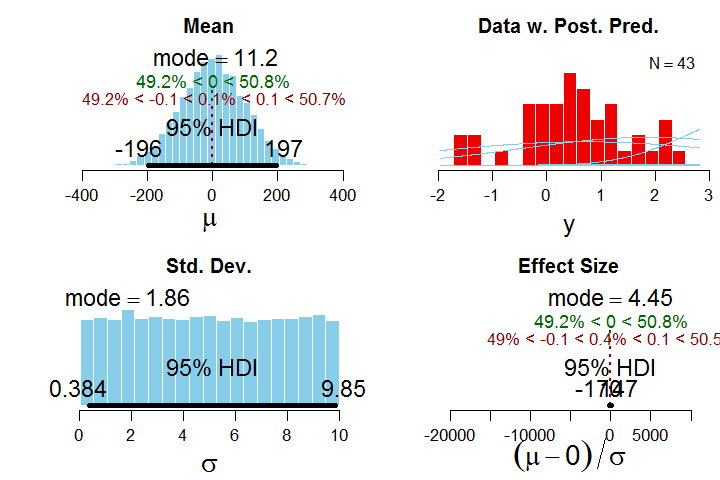Figure 4. Prior with unif(0,10) on sigma. Effect size is replotted in Figure 5. Compare with Figure 2.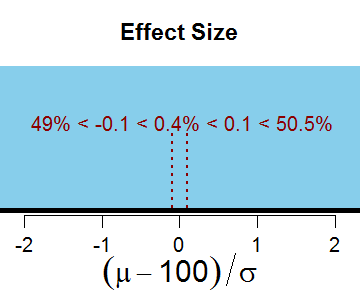Figure 5. Effect size replotted from Figure 4, using unif(0,10) on sigma.

The resulting posterior distribution looks like this: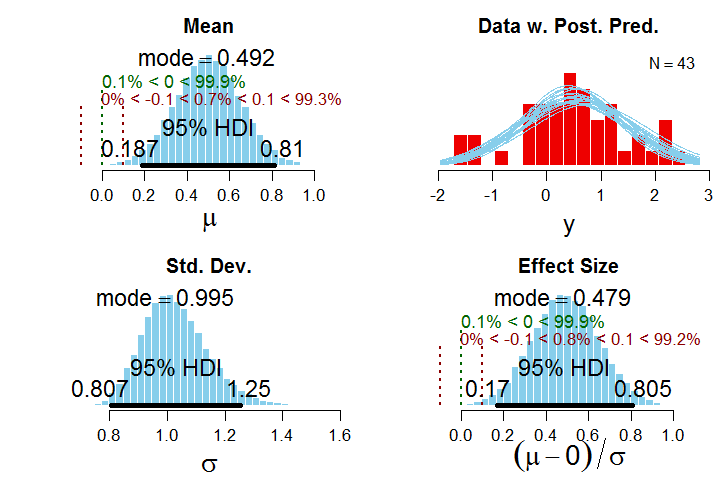Figure 6. Posterior when using unif(0,10) prior on sigma.
Compare the posterior in Figure 6 with the posterior in Figure 1. You will see they are basically identical. In other words, the 95% HDIs have barely changed at all, and decisions based on HDI and ROPE are identical, and the probability statements are identical.

But the BF for effect size is rather different than before. Now it is 0.8% / 0.4%, which is to say that the probability of the null hypothesis has gone up, i.e., this is a BF that leans in favor of the null hypothesis. Thus, a less vague prior on sigma has affected the implied prior on the effect size, which, of course, strongly affected the BF on effect size.

To summarize so far, a change in the breadth of the prior on sigma had essentially no effect on the HDIs of the posterior distribution, but had a big effect on the BF for the effect size while having no effect on the BF for mu.

Proponents of BFs will quickly point out that the priors used here are not well calibrated, i.e., they are too wide, too diluted. Instead, an appropriate use of BFs demands a well calibrated prior. (Proponents of BFs might even argue that an appropriate use of BFs would parameterize differently, focusing on effect size and sigma instead of mu and sigma.) I completely agree that the alternative prior must be meaningful and appropriate (again, see Ch. 12 of DBDA2E, or this article, or Appendix D of this article) and that the priors used here might not satisfy those requirements for a useful Bayes factor.

But there are still two take-away messages:
First, the BF for the mean (mu) need not lead to the same conclusion as the BF for the effect size unless the prior is set up just right.
Second, the posterior distribution on mu and effect size is barely affected at all by big changes in the vagueness of the prior, unlike the BF.

1.# Here is the R script I used to generate the graphs in the blog post:

# Generate the data:
set.seed(47405)
y = rnorm(43)
y = (y-mean(y))/sd(y)
y = y + 0.5
myData = y

# Specify filename root and graphical format for saving output.
fileNameRoot = "BFmeanBFeffSz-"
graphFileType = "png"

# Load the relevant model into R's working memory:
source("Jags-Ymet-Xnom1grp-Mnormal.R")

# Generate the MCMC chain:
mcmcCoda = genMCMC( data=myData , numSavedSteps=20000 , saveName=fileNameRoot )

# Display posterior information:
plotMCMC( mcmcCoda , data=myData ,
compValMu=0.0 ,
ropeMu=c(-0.1,0.1) ,
ropeEff=c(-0.1,0.1) ,
pairsPlot=TRUE , showCurve=FALSE ,
saveName=fileNameRoot , saveType=graphFileType )

# Plot of effect size only:
mcmcMat = as.matrix( mcmcCoda )
effSz = ( mcmcMat[,"mu"] - 0 ) / mcmcMat[,"sigma"]
openGraph(width=3.0,height=2.5)
par( mar=c(3.5,0.5,2.5,0.5) , mgp=c(2.25,0.7,0) )
plotPost( effSz , xlim=c(-2.0,2.0) , ROPE=c(-0.1,0.1) ,
main="Effect Size" , xlab=expression((mu-100)/sigma) )

# More details, not displayed in blog post:

# Display diagnostics of chain, for specified parameters:
parameterNames = varnames(mcmcCoda) # get all parameter names
for ( parName in parameterNames ) {
diagMCMC( codaObject=mcmcCoda , parName=parName ,
saveName=fileNameRoot , saveType=graphFileType )
}

# Get summary statistics of chain:
summaryInfo = smryMCMC( mcmcCoda ,
compValMu=0.0 ,
ropeMu=c(-0.1,0.1) ,
ropeEff=c(-0.1,0.1) ,
saveName=fileNameRoot )
show(summaryInfo)

2.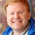What's the *argument* here? "First, the BF for the mean (mu) need not lead to the same conclusion as the BF for the effect size unless the prior is set up just right. Second, the posterior distribution on mu and effect size is barely affected at all by big changes in the vagueness of the prior, unlike the BF."

I'm missing some outline of a theory that says why this is "bad" or "wrong" (at least given the completely inappropriate priors you've used here), which seems to be the implication. But that can't be your argument, because that would invalidate Bayes' theorem and would be thus be self-contradictory.

3.A point I left tacit, but which was on my mind when I wrote the post, was that in frequentist tests of mean or effect size, the result is the same either way. That is, the p value for a test of the mean is the same as the p value for a test of the effect size. I think that most people (because of their frequentist training) think of the effect size as redundant with the mean, merely re-scaled. So it can be surprising that the BFs for mean and effect size can be different.

But even within the Bayesian framework, the marginal posterior distributions on the mean and effect size tend to be pretty commensurate, leading to pretty much the same conclusions (as long as the ROPEs are consistent). So it can be surprising that the BFs for mean and effect size can be different.

4.I think it says something very deep about Bayesian statistics (and inference in general) that the posterior and Bayes factor can be sensitive to different things. They're different quantities (the Bayes factor being evidence, and the posterior being a conclusion in light of the evidence) so one, in general, wouldn't expect them to behave in the same way. Since Bayes factors underly every Bayesian calculation -- even of posteriors for parameter estimation -- there's no doing without them.

The sensitivity of the evidence to background assumptions has important philosophical implications, and I think it's important that we not portray it as "undesirable."

5.Bayer Bless the posterior predictive distribution!

6.Dear John,
First, thanks for the great work ! I enjoy diving into Bayesian, which become my main occupation for the last few years. Yet, I am confused by two things with which I hope you can help me:
- Why is BF in this post discussed as a ratio between posterior and prior within ROPE (when performing a test on the mean) and not between the posterior odds and prior odds (unless, of course, the posterior of the null hypothesis and the prior of the null hipothesis are assumed to be 1 within ROPE). I'm definetly missing something.
- second thing is the most "burning question": How do we choose ROPE? I found in your article "Bayesian analysis" (2010) where it's indicated as "arbitrarily small to solve the technical false alarm problem". Is there a rule of thumb in chooseing ROPE for time series data? perhaps the standard deviation of the noise?